# Olympiad Test: Pattern - 2

## 10 Questions MCQ Test Science Olympiad Class 7 | Olympiad Test: Pattern - 2

Description
Attempt Olympiad Test: Pattern - 2 | 10 questions in 20 minutes | Mock test for Class 7 preparation | Free important questions MCQ to study Science Olympiad Class 7 for Class 7 Exam | Download free PDF with solutions
QUESTION: 1

### Directions: Choose the appropriate number which follow the given pattern.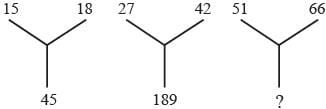Solution: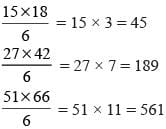QUESTION: 2

### Directions: Choose the appropriate number which follow the given pattern.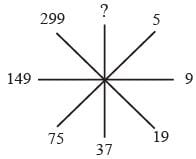Solution:

5 × 2 – 1 = 9;
9 × 2 + 1 = 19
19 × 2 – 1 = 37
37 × 2 + 1 = 75
75 × 2 – 1 = 149
149 × 2 + 1 = 299
299 × 2 – 1 = 597

QUESTION: 3

### Directions: Choose the appropriate number which follow the given pattern.​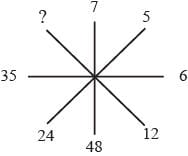Solution:

72 – 1 = 48
52 – 1 = 24
62 – 1 = 35
122 – 1 = 143

QUESTION: 4

Directions: Choose the appropriate number which follow the given pattern.​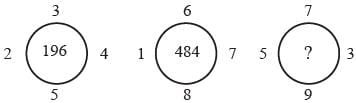Solution:

3 + 2 + 5 + 4 = 14 → 142 = 196
6 + 1 + 8 + 7 = 22 → 222 = 484
5 + 9 + 3 + 7 = 24 → 242= 576

QUESTION: 5

Look carefully for the pattern, and then choose which pair of numbers comes next:

9 11 33 13 15 33 17

Solution:

In this alternating repetition series, a random number, 33, is interpolated every third number into a simple addition series, in which each number increases by 2.

QUESTION: 6

Directions: Choose the appropriate number which follow the given pattern.​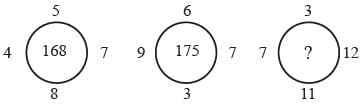Solution:

(4 + 8 + 7 + 5) × 7 = 24 × 7 = 168
(9 + 3 + 7 + 6) × 7 = 25 × 7 = 175
(7 + 11 + 12 + 3) = 33 × 7 = 231

QUESTION: 7

Directions: Choose the appropriate number which follow the given pattern.​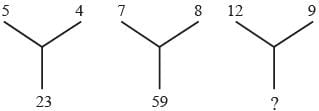Solution:

(5 × 4) + 3 = 23
(7 × 8) + 3 = 59
(12 × 9) + 3 = 111

QUESTION: 8

Directions: Choose the appropriate number which follow the given pattern.​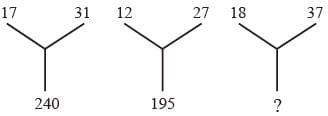Solution:

(17 + 31) × 5 = 240
(12 + 27) × 5 = 195
(18 + 37) × 5 = 275

QUESTION: 9

Directions: Choose the appropriate number which follow the given pattern.​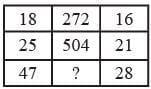Solution:

(18 – 1) × 16 = 272
(25 – 1) × 21 = 504
(47 – 1) × 28 = 1288

QUESTION: 10

​Directions: Choose the appropriate number which follow the given pattern.​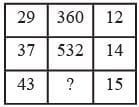Solution:

(29 + 1) × 12 = 360
(37 + 1) × 14 = 532
(43 + 1) × 15 = 660Use Code STAYHOME200 and get INR 200 additional OFF Use Coupon Code